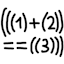#Prayer brackets

## Introduction

If the code doesn’t work, add more brackets. It’s a fundamental programming technique that beginners acquire quickly without consciously realizing, and one that seasoned programmers apply without a second thought. But when I encounter over-bracketed code like this, I can’t help but cringe:

```if (((((foo * bar) + alpha > 0) && (abs(omega[(i + j)]) == 3))) || (x < y))
((beta->gamma)[i])->delta = (f(zeta + 1) + g(pi));```

This madness of littering unnecessary brackets everywhere must stop. The code above simplifies to:

```if (foo * bar + alpha > 0 && abs(omega[i + j]) == 3 || x < y)
beta->gamma[i]->delta = f(zeta + 1) + g(pi);```

I believe this behavior grows over time as a programmer encounters bracket-related compile/logic errors over and over again, and as a result chooses to write code in a conservative way.

## The solution

First, let’s review the underlying concepts. Brackets are necessary because we use infix operators to write our expression trees. (Brackets are unnecessary for prefix and postfix notation.) The usage of brackets and infix operators in programming languages is carried over from standard math notation. The system is just an extension of PEDMAS learned in elementary school, but with about a dozen operators and a dozen levels of precedence.

The rationale for having operator precedence (order of operations) is to reduce the use of brackets in common cases. For example, times (`*`) is defined to have a higher precedence than plus (`+`). Therefore, in the expression `(a * b) + c`, the brackets are redundant and the expression is equivalent to `a * b + c`. Similarly, `==` has higher precedence than `&&`, which in turn has higher precedence than `||`. Knowing these facts means we can rewrite `((a == b) && c) || d` as simply `a == b && c || d`.

So, what is the solution to the problem of writing too many unnecessary brackets in the code? Consult your programming language’s documentation. Don’t guess what the operator precedence is, and don’t give up and add brackets for safety. Take a moment to read the documentation to find out exactly in what situations brackets are necessary and in what situations they are unnecessary. Here are some handy links to operator precedence tables for a select few popular languages: C/C++, Java, Python, JavaScript, Haskell.

## On the other hand

Writing clear code is not an exact science with clear-cut right-or-wrong answers in every situation, and there are some cases where using more brackets than necessary is acceptable.

For C preprocessor macros, you might see code like this: `#define min(a,b) ((a)<(b)?(a):(b))`. It almost looks like you can simplify it to `#define min(a,b) a<b?a:b`, but this is not true because the preprocessor handles tokens, not evaluated expression values.

For some infrequently used operators such as the bitwise operators, it might be hard to remember their order of precedence, and different languages may treat them differently. So although all the brackets are redundant in the following example expression in C, they are still generally a good idea: `((x << 8) & 0xF00) | (y & 0xFF)`.

## Notes

• In C (and various languages inheriting its syntax, such as Java), it’s unfortunate that `&` has lower precedence than `==`. For example, to test against a bit mask, one might be tempted to write code like `if (x & 1 == 0)`, but it is interpreted as `if (x & (1 == 0))`. In C, this snippet of code compiles and runs but does not behave as expected. In Java, this is a compile-time error due to type incompatibility. In both these languages, the correct code is `if ((x & 1) == 0)`. But curiously, Python uses a more natural precedence, and this bracket-less code is still correct: `if x & 1 == 0: ...`.

• The title and first sentence are kind of an allusion to the notion of “If it doesn’t work, pray harder”.

• : In particular I mean round brackets, which are formally called parentheses.

• : Such as reverse Polish notation (RPN).

• : In case the code is expected to be translated to a different programming language sometime.